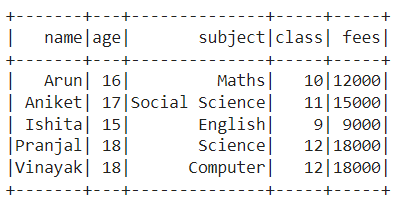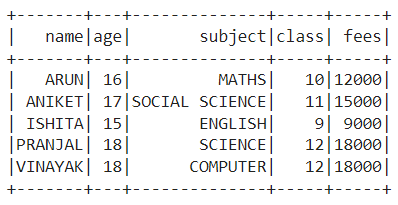Open in App
Not now

# Apply a transformation to multiple columns PySpark dataframe

• Last Updated : 30 Jan, 2023

In this article, we are going to learn how to apply a transformation to multiple columns in a data frame using Pyspark in Python.

The API which was introduced to support Spark and Python language and has features of Scikit-learn and Pandas libraries of Python is known as Pyspark. While using Pyspark, you might have felt the need to apply the same function whether it is uppercase, lowercase, subtract, add, etc. to apply to multiple columns. This is possible in Pyspark in not only one way but numerous ways. In this article, we will discuss all the ways to apply a transformation to multiple columns of the PySpark data frame.

## Method 1: Using reduce function

An aggregate action function that is used to calculate the min, the max and the total of elements in a dataset is known as reduce function. In this method, we will import the CSV file or create the dataset and then apply a transformation using reduce function to the multiple columns of the uploaded or the created data frame.

### Stepwise Implementation:

Step 1: First, import the required libraries, i.e. SparkSession, reduce, col, and upper. The SparkSession library is used to create the session, while reduce applies a particular function passed to all of the list elements mentioned in the sequence. The col is used to get the column name, while the upper is used to convert the text to upper case. Instead of upper, you can use any other function too that you want to apply on each row of the data frame.

```from pyspark.sql import SparkSession
from functools import reduce
from pyspark.sql.functions import col, upper```

Step 2: Now, create a spark session using the getOrCreate function.

`spark_session = SparkSession.builder.getOrCreate()`

Step 3: Then, read the CSV file or create the data frame using the createDataFrame function.

```data_frame=csv_file = spark_session.read.csv('#Path of CSV file',
sep = ',', inferSchema = True, header = True)```

or

```data_frame=spark_session.createDataFrame([(column_1_data), (column_2_data), (column_3_data)],
['column_name_1', 'column_name_2', 'column_name_3'])```

Step 4: Next, apply a particular function passed as an argument to all the row elements of the data frame using reduce function.

```updated_data_frame = (reduce(lambda traverse_df,
col_name: traverse_df.withColumn(col_name, upper(col(col_name))),
data_frame.columns, data_frame))```

Step 5: Finally, display the updated data frame in the previous step.

`updated_data_frame.show()`

Example:

In this example, we have uploaded the CSV file (link), i.e., basically a data set of 5*5 as follows:Then, we used the reduce function to apply a transformation to multiple columns ‘name‘ and ‘subject‘ of the Pyspark data frame uppercase through the function upper.

## Python3

 `# Python program to apply a transformation to multiple ``# columns of PySpark dataframe using reduce function`` ` `# Import the SparkSession, reduce, col and upper libraries``from` `pyspark.sql ``import` `SparkSession``from` `functools ``import` `reduce``from` `pyspark.sql.functions ``import` `col, upper`` ` `# Create a spark session using getOrCreate() function``spark_session ``=` `SparkSession.builder.getOrCreate()`` ` `# Read the CSV file``data_frame``=``csv_file ``=` `spark_session.read.csv(``        ``'/content/student_data.csv'``,``        ``sep ``=` `','``, inferSchema ``=` `True``,``        ``header ``=` `True``)`` ` `# Apply a transformation to multiple columns of``# PySpark dataframe using reduce function``updated_data_frame ``=` `(``reduce``(``lambda` `traverse_df,``  ``col_name: traverse_df.withColumn(col_name,``  ``upper(col(col_name))), data_frame.columns,``                         ``data_frame))`` ` `# Show the updated data frame``updated_data_frame.show()`

Output:## Method 2: Using for loop

A particular way of iterating over a sequence, i.e., a list, a tuple, a dictionary, a set, or a string) is known as for loop. In this method, we will import the CSV file or create the dataset and then apply a transformation using for loop to the multiple columns of the uploaded or the created data frame.

### Stepwise Implementation

Step 1: First, import the required libraries, i.e. SparkSession, reduce, col, and upper. The SparkSession library is used to create the session. The col is used to get the column name, while the upper is used to convert the text to upper case. Instead of upper, you can use any other function too that you want to apply on each row of the data frame.

```from pyspark.sql import SparkSession
from pyspark.sql.functions import col, upper```

Step 2: Now, create a spark session using the getOrCreate function.

`spark_session = SparkSession.builder.getOrCreate()`

Step 3: Then, read the CSV file or create the data frame using the createDataFrame function.

```data_frame=csv_file = spark_session.read.csv('#Path of CSV file',
sep = ',', inferSchema = True, header = True)```

or

```data_frame=spark_session.createDataFrame([(column_1_data), (column_2_data), (column_3_data)],
['column_name_1', 'column_name_2', 'column_name_3'])```

Step 4: Next, create a for loop to traverse all the elements and convert it to uppercase.

```for col_name in data_frame.columns:
data_frame = data_frame.withColumn(col_name, upper(col(col_name)))```

Step 5: Finally, display the updated data frame in the previous step.

`data_frame.show()`

### Example:

In this example, we have uploaded the CSV file (link), i.e., basically a data set of 5*5 as follows:Then, we used the for loop to apply a transformation to multiple columns ‘name‘ and ‘subject‘ of the Pyspark data frame uppercase through the function upper.

## Python3

 `# Python program to apply a transformation to multiple ``# columns of PySpark dataframe using for loop`` ` `# Import the SparkSession, col and upper libraries``from` `pyspark.sql ``import` `SparkSession``from` `pyspark.sql.functions ``import` `col, upper`` ` `# Create a spark session using getOrCreate() function``spark_session ``=` `SparkSession.builder.getOrCreate()`` ` `# Read the CSV file``data_frame``=``csv_file ``=` `spark_session.read.csv(``          ``'/content/student_data.csv'``,``          ``sep ``=` `','``, inferSchema ``=` `True``,``          ``header ``=` `True``)`` ` `# Apply a transformation to multiple ``# columns of PySpark dataframe using for loop``for` `col_name ``in` `data_frame.columns:``    ``data_frame ``=` `data_frame.withColumn(col_name,``                           ``upper(col(col_name)))`` ` `# Show the updated data frame``data_frame.show()`

Output:## Method 3: Using list comprehension

A shorter way of creating a new list based on the values of an existing list is known as list comprehension. In this method, we will import the CSV file or create the dataset and then apply a transformation using list comprehension to the multiple columns of the uploaded or the created data frame.

### Stepwise Implementation:

Step 1: First, import the required libraries, i.e. SparkSession,  col, and upper. The SparkSession library is used to create the session. The col is used to get the column name, while the upper is used to convert the text to upper case. Instead of upper, you can use any other function too that you want to apply on each row of the data frame.

```from pyspark.sql import SparkSession
from pyspark.sql.functions import col, upper```

Step 2: Now, create a spark session using the getOrCreate function.

`spark_session = SparkSession.builder.getOrCreate()`

Step 3: Then, read the CSV file or create the data frame using the createDataFrame function.

```data_frame=csv_file = spark_session.read.csv('#Path of CSV file',
sep = ',', inferSchema = True, header = True)```

or

```data_frame=spark_session.createDataFrame([(column_1_data), (column_2_data), (column_3_data)],
['column_name_1', 'column_name_2', 'column_name_3'])```

Step 4: Next, create a list comprehension to traverse all the elements and convert it to uppercase.

```updated_data_frame = data_frame.select(
*[upper(col(col_name)).name(col_name) for col_name in data_frame.columns])```

Step 5: Finally, display the updated data frame in the previous step.

`updated_data_frame.show()`

### Example:

In this example, we have uploaded the CSV file (link), i.e., basically a data set of 5*5 as follows:Then, we used the list comprehension to apply a transformation to multiple columns ‘name‘ and ‘subject‘ of the Pyspark data frame uppercase through the function upper.

## Python3

 `# Python program to apply a transformation to multiple ``# columns of PySpark dataframe using list comprehension`` ` `# Import the SparkSession, col and upper libraries``from` `pyspark.sql ``import` `SparkSession``from` `pyspark.sql.functions ``import` `col, upper`` ` `# Create a spark session using getOrCreate() function``spark_session ``=` `SparkSession.builder.getOrCreate()`` ` `# Read the CSV file``data_frame``=``csv_file ``=` `spark_session.read.csv(``      ``'/content/student_data.csv'``,``      ``sep ``=` `','``, inferSchema ``=` `True``,``      ``header ``=` `True``)`` ` `# Apply a transformation to multiple columns of ``# PySpark dataframe using list comprehension``updated_data_frame ``=` `data_frame.select(`` ``*``[upper(col(col_name)).name(col_name) ``for` `col_name ``in` `data_frame.columns])`` ` `# Show the updated data frame``updated_data_frame.show()`

Output:My Personal Notes arrow_drop_up